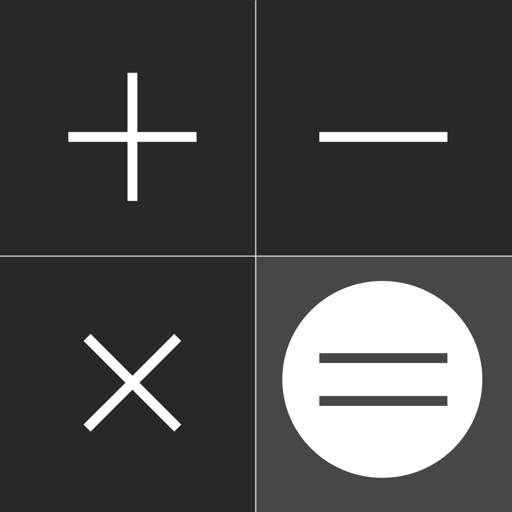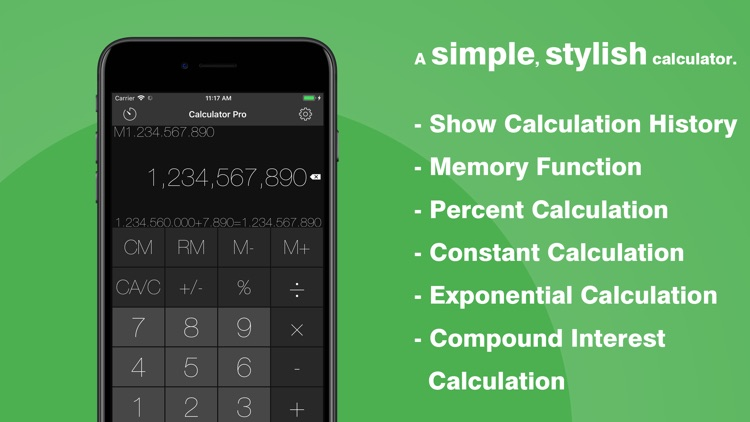## A simple, stylish calculator# Calculator【計算機】- Simple計算機 Pro

by woodsmall inc.A simple, stylish calculator.### App Details

Version
1.4.2
Rating
(5)
Size
39Mb
Genre
Productivity Finance
Last updated
July 13, 2019
Release date
September 22, 2013

### App Store Description

A simple, stylish calculator.
One use of this free app, and you’ll never want to be without it.

Percent calculations make calculating sales tax easy.

Calculator supports the following features.
[Percent Calculation]
500×5%25
500÷5%10000
500-5%475
500+5%525

[Constant Calculation]
After inputting the desired number, tap the desired operator twice to set it as the constant.
Ex) When you want to constantly add 100
100++
1000=1100
2000=2100

[Exponential Calculation]
5××=25
=125
=625

[Compound Interest Calculation]
Ex) When calculating the yearly maturity on 0.5% (after tax) of \$10,000 deposited yearly.
1.005××
10000=10050
=10100.25
=10150.751

Calculator does not support function operations.
It uses Sharp-style calculation formulas.

[Main Functions]
- Input up to 12 digits.